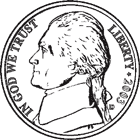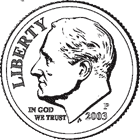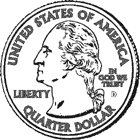Create a new printableProbability
Math Worksheets

Sample - Click above to make a new math worksheet (PDF).
 Name _____________________________Date ___________________
Probability
Write whether each event is certain, likely, unlikely, or impossible.

1.
 A glass jar contains a total of 36 marbles. The jar has purple and red marbles. There are 7 red marbles. Describe the probability of picking a yellow marble.2.
 The following coins are put in a bag:Describe the probability of picking a dime.3.
 A glass jar contains 17 red marbles. Describe the probability of picking a red marble.4 * This is a pre-made sheet.Use the link at the top of the page for a printable page.
5.
 A glass jar contains a total of 33 marbles. The jar has red and green marbles. There are 29 red marbles. Describe the probability of picking a green marble.6.
 The following coins are put in a bag:Describe the probability of picking a quarter.7.
 The following coins are put in a bag:Describe the probability of picking a nickel.8.
 A number from the following list is chosen at random:  17, 83, 63, 9, 85, 7, 13, and 3. Describe the probability of picking an odd number.9.
 The following coins are put in a bag:Describe the probability of picking a quarter.10.
 A glass jar contains 15 green marbles. Describe the probability of picking a purple marble.